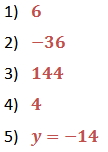Evaluate Algebraic Expressions

Evaluate Algebraic Expressions

To evaluate an algebraic expression means to –
• replace the variable(s) in the expression with the number value(s) given,
• place the given values in parentheses,
• simplify the expression using the order of operations, and
• determine the end result.

Example

Evaluate  4(x2 + 5x) - 2y, given that x = 2 and y = -3

Substitute 2 for x and -3 for y in the given expression:
4[(2)2 + 5 · (2)] - [2( -3)]

Simplify using the order of operations:
4[4 + 10] - [-6]
4  - [-6]
56 + 6
62

Practice

Substitute the given values for the variables to evaluate each expression.

1)  Evaluate  3(x2 + 4x) - 3y when x = -5 and y = 3

2)  Evaluate x2y  for x = -3 and y = -4

3)  Evaluate  (b - d)2  when b = 9 and d = -3

4)  Evaluate m4 + 2m3 - m2 + 8 for m = -2

5)  Evaluate y =  3x + 1 for x = -5